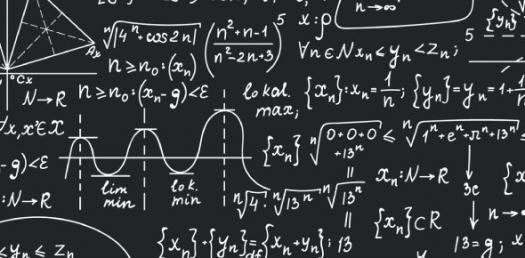# 1st And 2nd Grade Math Questions! Trivia Quiz

Approved & Edited by ProProfs Editorial Team
The editorial team at ProProfs Quizzes consists of a select group of subject experts, trivia writers, and quiz masters who have authored over 10,000 quizzes taken by more than 100 million users. This team includes our in-house seasoned quiz moderators and subject matter experts. Our editorial experts, spread across the world, are rigorously trained using our comprehensive guidelines to ensure that you receive the highest quality quizzes.
| By Jszypula
J
Jszypula
Community Contributor
Quizzes Created: 1 | Total Attempts: 1,114
Questions: 10 | Attempts: 1,124SettingsUnderstanding math can be a challenging subject and the 1st and 2nd grade math questions trivia quiz below has been prepared to give students the much needed practice so that they can refresh their understanding on how some of the math problems they have learnt about in class are solved. How about you give it a go and get to see which problems are a challenge for you. All the best!.

• 1.

### Lauren has 7 cards. Ryan has 10 cards. How many cards do they have altogether?

• A.

17

• B.

7

• C.

5

• D.

10

A. 17
Explanation
Lauren has 7 cards and Ryan has 10 cards. To find out how many cards they have altogether, we need to add the number of cards each of them has. Adding 7 and 10 gives us a total of 17 cards.

Rate this question:

• 2.

• A.

99

• B.

12

• C.

5

• D.

11

D. 11
• 3.

### If Brad had 10 cards but gave 7 of them to Ryan. How many cards does Brad have now?

3 cards
3
Explanation
Brad had 10 cards initially, but he gave 7 of them to Ryan. Therefore, the number of cards Brad has now can be calculated by subtracting the cards given to Ryan from the initial number of cards Brad had. Hence, Brad now has 3 cards.

Rate this question:

• 4.

### 14-7

• A.

7

• B.

10

• C.

5

• D.

6

• E.

14

A. 7
Explanation
The given expression is a subtraction problem. Subtracting 7 from 14 gives us the answer of 7.

Rate this question:

• 5.

• A.

5

• B.

7

• C.

9

• D.

3

B. 7
• 6.

### 5+8=13

• A.

True

• B.

False

A. True
Explanation
The given statement "5+8=13" is false. The correct answer is False.

Rate this question:

• 7.

### 13-7

• A.

5

• B.

7

• C.

4

• D.

6

D. 6
Explanation
The given expression is 13-7, which equals 6.

Rate this question:

• 8.

### If you ate 9 cookies an ate 11 more how many did you eat?

• A.

• B.

• C.

• D.

Explanation
If you ate 9 cookies and then ate 11 more, you would have eaten a total of 20 cookies.

Rate this question:

• 9.

• A.

8

• B.

15

• C.

10

• D.

9